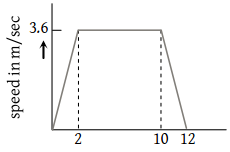A lift is going up. The total mass of the lift and the passenger is 1500 kg. The variation in the speed of the lift is as given in the graph. The tension in the rope pulling the lift at t = 11th sec will be(1) 17400 N

(2) 14700 N

(3) 12000 N

(4) Zero

High Yielding Test Series + Question Bank - NEET 2020

Difficulty Level:

A lift is going up. The total mass of the lift and the passenger is 1500 kg. The variation in the speed of the lift is as given in the graph. The height to which the lift takes the passenger is(1) 3.6 meters

(2) 8 meters

(3) 1.8 meters

(4) 36 meters

High Yielding Test Series + Question Bank - NEET 2020

Difficulty Level:

A player caught a cricket ball of mass 150 gm moving at a rate of 20 m/s. If the catching process be completed in 0.1 s, then the force of the blow exerted by the ball on the hands of the player is

(1) 0.3 N

(2) 30 N

(3) 300 N

(4) 3000 N

Concept Questions :-

Newton laws
High Yielding Test Series + Question Bank - NEET 2020

Difficulty Level:

A rocket has a mass of 100 kg. 90% of this is fuel. It ejects fuel vapours at the rate of 1 kg/sec with a velocity of 500 m/sec relative to the rocket. It is supposed that the rocket is outside the gravitational field. The initial upthrust on the rocket when it just starts moving upwards is

(1) Zero

(2) 500 N

(3) 1000 N

(4) 2000 N

Concept Questions :-

Variable mass system
High Yielding Test Series + Question Bank - NEET 2020

Difficulty Level:

In which of the following cases forces may not be required to keep the

(1) Particle going in a circle

(2) Particle going along a straight line

(3) The momentum of the particle constant

(4) Acceleration of the particle constant

Concept Questions :-

Newton laws
High Yielding Test Series + Question Bank - NEET 2020

Difficulty Level:

A ball of mass 150g starts moving with an acceleration of 20 m/s2. When hit by a force, which acts on it for 0.1 sec. The impulsive force is

(1) 0.5 N-s

(2) 0.1 N-s

(3) 0.3 N-s

(4) 1.2 N-s

Concept Questions :-

Newton laws
High Yielding Test Series + Question Bank - NEET 2020

Difficulty Level:

A body, whose momentum is constant, must have constant

(1) Force

(2) Velocity

(3) Acceleration

(4) All of these

Concept Questions :-

Newton laws
High Yielding Test Series + Question Bank - NEET 2020

Difficulty Level:

Rocket engines lift a rocket from the earth surface because hot gas with high velocity

(1) Push against the earth

(2) Push against the air

(3) React against the rocket and push it up

(4) Heat up the air which lifts the rocket

Concept Questions :-

Variable mass system
High Yielding Test Series + Question Bank - NEET 2020

Difficulty Level:

A force of 50 dynes is acted on a body of mass 5 g which is at rest for an interval of 3 seconds, then impulse is

(1) $0.15×{10}^{-3}\text{\hspace{0.17em}}Ns$

(2) $0.98×{10}^{-3}\text{\hspace{0.17em}}Ns$

(3) $1.5×{10}^{-3}\text{\hspace{0.17em}}Ns$

(4) $2.5×{10}^{-3}\text{\hspace{0.17em}}Ns$

Concept Questions :-

Newton laws
High Yielding Test Series + Question Bank - NEET 2020

Difficulty Level:

A bullet of mass 0.1 kg is fired with a speed of 100 m/sec, the mass of gun is 50 kg. The velocity of recoil is

1. 0.2 m/sec

2. 0.1 m/sec

3. 0.5 m/sec

4. 0.05 m/sec

Concept Questions :-

Newton laws